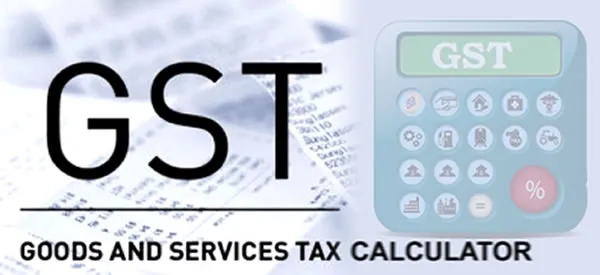Feet on the Ground, Books in the Cloud, Profits to the Moon...

# New Zealand 15% GST CalculatorHow to Correctly Calculate Goods & Services Tax Costs for New Zealand

These New Zealand GST calculators work out:

• The GST to add to an GST Exclusive amount
• The GST content of a GST Inclusive amount
• The original costs based just on the GST amount

## NZ Online GST Calculator

Enter any known amount into the appropriate box to calculate the other 2 figures.

• Add 15% GST to a GST Exclusive Price
• Find the GST component of a GST Inclusive Price
• Find GST Exclusive Price from full cost including GST
 Cost Excluding GST \$ GST Amount \$ Cost Including GST \$

## The mathematics behind calculating GST in NZ

Basic GST Calculations.

Goods and Services Tax in NZ is 15%

To add 15% GST on \$100

• \$100 x 15% = \$15 GST
• \$100 + \$15 GST = \$115 GST Inclusive.

A quick way to Add GST is to Multiply
the GST exclusive amount by 1.15

Examples Below:

• \$200 + GST = \$200 x 1.15
= \$230
• \$500 + GST = \$500 x 1.15
= \$575

### Subtracting GST

Inland Revenue (IRD) recommends the following formula to find the GST amount from a GST inclusive price.

• GST Inclusive Price X 3 ÷ 23 = GST Amount

Working backwards to find the GST and GST exclusive amounts from the total GST inclusive price.

• \$115 X 3 ÷ 23 = \$15 GST

To work out the GST exclusive amount simply subtract the GST from the GST inclusive amount to get the original GST exclusive cost.

• \$115 – \$15 GST = \$100

When using the correct formula, you can see the \$15 GST cost remains the same when adding GST on and finding the GST amount from the inclusive price.

#### Subtracting GST the wrong way!

Don't Just Remove 15%

Just subtracting 15% from the GST inclusive price won't work.

• 15% of \$115 = \$17.25
(not \$15)

To subtract 15% from our \$115 GST inclusive figure from above…

• \$115 – 15% = \$97.75
(not \$100)

## Like what you have read?

Contact Devine Accounting to book an initial consultation.